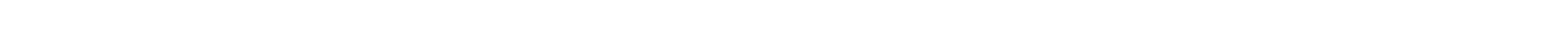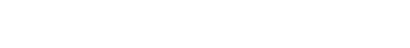## Machine Learning Tutorial

Linear Regression refers to an approach/algorithm that helps establish a linear relationship between the dependant and the independent variable. As the name indicates, it is a linear process, which means it is 2 dimensional, i.e. it has 2 variables associated with it. These variables have continuous values (in contrast to 0s and 1s in logistic regression). The word ‘regression’ refers to finding relationship between two variables amongst which one is a dependant variable and the other one is independent. Linear Regression is one of the most widely used and well understood algorithm in the field of statistics and Machine Learning. How can this relationship be established? In simple words, it goes like this- we will be provided with a basic linear equation, say y = 3x-1. Here ‘y’ is considered to be the dependant variable (since it depends on the value of x) and ‘x’ (trivially) is the independent variable. This means, as and when ‘x’ changes, the value of ‘y’ keeps changing according to the above-mentioned linear equation. Different values for ‘x’ are supplied, which helps calculate various values for ‘y’. The values for ‘x’ and ‘y’ have been shown in a table below: XY122538411514617720These values are plotted on a graph and we try to fit all these points (or most of them) to a straight line. During the process of fitting these values to a straight line, we try and grab most of the points whose vertical distance from the straight line (that is being fit) is minimum. Some points don’t make it on the straight line since they don’t contribute in forming a straight line. These are the ones whose vertical distance from the straight line isn’t the smallest. The idea is to grab all the points in the graph and fit them on a straight line that have minimum vertical distance from the line. Below is an example illustrating the same: When the number of points that don’t contribute to fitting a straight line are more in comparison to the ones that contribute to fitting the line, it is considered that the ‘prediction error’ is more. The ‘error’ basically refers to the shortest distance (vertical distance) between the line and the point. From the above graph, it can be observed that points 1,2,3 and 4 beginning from the bottom left corner don’t really fit the line, and don’t contribute to forming the straight line. When such a linear regression model is trained, it helps calculate an attribute called ‘cost function’ that helps in measuring the ‘Root Mean Squared Error’ or RMSE in short. RMSE basically gives the difference between the values that are predicted and the input values. These values are then normalized by squaring them so as to remove any negative values and calculating the average of these values (i.e dividing them by the total number of observations) and taking the square root of this value. The resultant is a single number that is used to understand how well the regression algorithm has predicted output for a given input value and how close it is to the actual output. The ‘cost function’ needs to be minimal, thereby corresponding to a minimum difference between the actual value and the predicted value. Gradient Descent Gradient descent is an optimization algorithm which is used to minimize the cost function by providing the right values for the parameters used in the linear function (the gradient is actually a derivative of the loss). This doesn’t happen in a single step, but takes multiple steps to finally arrive at a value which is minimum, and going further from there would lead to no other better value. Inferences that can be made with the help of the gradient descent: If the gradients obtained are positive, the loss increases when the data element’s value is increased by a small amount and the loss reduces when the data element’s value is decreased by a small amount. If the gradients obtained are negative, the loss decreases when the data element’s value is increased by a small amount and the loss increases when the data element’s value is decreased by a small amount. Stochastic Gradient Descent is another variation of Gradient Descent whose ultimate goal is to minimize the cost function. Implementation in Python In Python, Linear regression can be implemented using the scikit-learn library. import numpy as np  import matplotlib.pyplot as plt  from sklearn.linear_model import LinearRegression  from sklearn.metrics import mean_squared_error, r2_score  #A random data set is generated  np.random.seed(0)  x = np.random.rand(100, 1)  y = -3.5 + 5.19* x + np.random.rand(100, 1)  #The model is initialized  regression_model = LinearRegression()  The data is fit on the model, with the help of training regression_model.fit(x, y)  The output is predicted y_predicted = regression_model.predict(x)  The model built is evaluated using mean squared error parameter rmse = mean_squared_error(y, y_predicted)  r2 = r2_score(y, y_predicted)  print("The slope value is: ", regression_model.coef_)  print("The intercept is: ", regression_model.intercept_)  print("The Root mean squared error is: ", rmse)  #The data is visualized usign the matplotlib library  plt.scatter(x, y, s=8)  plt.xlabel('X axis')  plt.ylabel('Y axis')  The values that are predicted plt.plot(x, y_predicted, color='g') plt.show() Output: The slope value is: [[5.12655106]]  The intercept is: [-2.94191998]  The Root mean squared error is: 0.07623324582875007 Conclusion In this post, we understood the significance of Linear Regression and its implementation using Python.

# Linear Regression(Python Implementation)

Linear Regression refers to an approach/algorithm that helps establish a linear relationship between the dependant and the independent variable.

As the name indicates, it is a linear process, which means it is 2 dimensional, i.e. it has 2 variables associated with it. These variables have continuous values (in contrast to 0s and 1s in logistic regression). The word ‘regression’ refers to finding relationship between two variables amongst which one is a dependant variable and the other one is independent.

Linear Regression is one of the most widely used and well understood algorithm in the field of statistics and Machine Learning.

### How can this relationship be established?

In simple words, it goes like this- we will be provided with a basic linear equation, say y = 3x-1. Here ‘y’ is considered to be the dependant variable (since it depends on the value of x) and ‘x’ (trivially) is the independent variable. This means, as and when ‘x’ changes, the value of ‘y’ keeps changing according to the above-mentioned linear equation. Different values for ‘x’ are supplied, which helps calculate various values for ‘y’. The values for ‘x’ and ‘y’ have been shown in a table below:

XY
12
25
38
411
514
617
720

These values are plotted on a graph and we try to fit all these points (or most of them) to a straight line. During the process of fitting these values to a straight line, we try and grab most of the points whose vertical distance from the straight line (that is being fit) is minimum. Some points don’t make it on the straight line since they don’t contribute in forming a straight line. These are the ones whose vertical distance from the straight line isn’t the smallest. The idea is to grab all the points in the graph and fit them on a straight line that have minimum vertical distance from the line. Below is an example illustrating the same:When the number of points that don’t contribute to fitting a straight line are more in comparison to the ones that contribute to fitting the line, it is considered that the ‘prediction error’ is more. The ‘error’ basically refers to the shortest distance (vertical distance) between the line and the point.

From the above graph, it can be observed that points 1,2,3 and 4 beginning from the bottom left corner don’t really fit the line, and don’t contribute to forming the straight line.

When such a linear regression model is trained, it helps calculate an attribute called ‘cost function’ that helps in measuring the ‘Root Mean Squared Error’ or RMSE in short. RMSE basically gives the difference between the values that are predicted and the input values. These values are then normalized by squaring them so as to remove any negative values and calculating the average of these values (i.e dividing them by the total number of observations) and taking the square root of this value.

The resultant is a single number that is used to understand how well the regression algorithm has predicted output for a given input value and how close it is to the actual output. The ‘cost function’ needs to be minimal, thereby corresponding to a minimum difference between the actual value and the predicted value.

Gradient descent is an optimization algorithm which is used to minimize the cost function by providing the right values for the parameters used in the linear function (the gradient is actually a derivative of the loss). This doesn’t happen in a single step, but takes multiple steps to finally arrive at a value which is minimum, and going further from there would lead to no other better value.

### Inferences that can be made with the help of the gradient descent:

If the gradients obtained are positive, the loss increases when the data element’s value is increased by a small amount and the loss reduces when the data element’s value is decreased by a small amount.

If the gradients obtained are negative, the loss decreases when the data element’s value is increased by a small amount and the loss increases when the data element’s value is decreased by a small amount.

Stochastic Gradient Descent is another variation of Gradient Descent whose ultimate goal is to minimize the cost function.

### Implementation in Python

In Python, Linear regression can be implemented using the scikit-learn library.

import numpy as np
import matplotlib.pyplot as plt
from sklearn.linear_model import LinearRegression
from sklearn.metrics import mean_squared_error, r2_score
#A random data set is generated
np.random.seed(0)
x = np.random.rand(100, 1)
y = -3.5 + 5.19* x + np.random.rand(100, 1)
#The model is initialized
regression_model = LinearRegression()
The data is fit on the model, with the help of training regression_model.fit(x, y)
The output is predicted
y_predicted = regression_model.predict(x)
The model built is evaluated using mean squared error parameter rmse = mean_squared_error(y, y_predicted)
r2 = r2_score(y, y_predicted)
print("The slope value is: ", regression_model.coef_)
print("The intercept is: ", regression_model.intercept_)
print("The Root mean squared error is: ", rmse)
#The data is visualized usign the matplotlib library
plt.scatter(x, y, s=8)
plt.xlabel('X axis')
plt.ylabel('Y axis')
The values that are predicted plt.plot(x, y_predicted, color='g') plt.show() 

Output:

The slope value is: [[5.12655106]]
The intercept is: [-2.94191998]
The Root mean squared error is: 0.07623324582875007#### Conclusion

In this post, we understood the significance of Linear Regression and its implementation using Python.#### Vinu

After reading your article, I was amazed. I know that you explain it very well. And I hope that other readers will also experience how I feel after reading your article. Thanks for sharing.#### Johnson M

Good and informative article.#### Vinu

I enjoyed reading your articles. This is truly a great read for me. Keep up the good work!#### Vinu